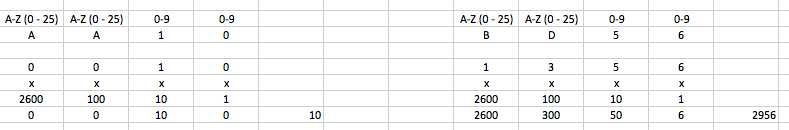Currently, addresses can be presented in two formats. One way of address presentation is created for being read by a person; in the future, we will call such a representation a textual representation or a textual format. However, inside of transactions, in the blockchain itself, etc. addresses can be presented differently. Another way of address presentation is binary one. It is recommended to use the text representation of the address wherever the user is supposed to work with it (interface display of the address, or when user sends the transaction to this address, etc.).

Example of address in textual representation:

``AA100000001677722412``

Example of an address in a binary representation in the form hex:

``800140000100000B``

Moreover, the addresses also can be public and private. Public addresses are available globally (then it is possible to make a transaction in another chain), and private addresses, which can be used only within a private chain.

## 1. Address structure in binary representation​

The address is actually 8 bytes of binary data. While encoding the address or in various transformations associated with it, the location of the BigEndian bits is used, i.e. the highest bits of the address on the left, the least significant bits of the address on the right. In binary form, the bits will have the following values:

``100GGGGG GGGGGGGG GGGBBBBB BBBBBBBB BBBBBBBB AAAAAAAA AAAAAAAA AAAAAAAA``

or:

``101BBBBB BBBBBBBB BBBBBBBB BBBBBBBB BBBBBBBB AAAAAAAA AAAAAAAA AAAAAAAA``

The three high-order bits indicate the type of the address.

• 101 - private Any other value in the three most significant bits makes the address invalid. The remaining bits have the following meaning: G - the public address group ID.B - the identifier of the address block.A - the wallet identifier in the block.

## 2. Converting an address from a text representation to a binary one​

In the textual format, private addresses have a length of 18 characters while public addresses have 20 characters length. Moreover, they are also converted in different ways. If you want to choose proper algorithm for this transformation, first you need to define public or private address you are dealing with. To do this, look at the length:

• 18 characters - private (example of private address: 00000000FE00007DF0)
• 20 characters - public (example of public address: AA100000001677722412) We break the address into parts, each of which is converted into a binary form according to different rules. The public address consists of the following parts:
``AADDLLLLLLLLLLLLLLCC``

Aletters of the Latin alphabet; Ddigits, together AADD encode the group identifier; Ldigits, in this part the identifiers of the addresses block and the identifiers of a wallet block are coded; Cthe lowest part of the address checksum (the last 2 digits of the checksum written in decimal form); Let's look at how each part is coded. As already mentioned, the first 4 characters of the address in the text representation encode the identifier of the group, but the letters and digits are transformed according to different rules. The letters (the first 2 characters) represent a number in the 26-digit number system (ie A-0, B-1, C-2, ..., Z-25). Numbers (ie the next 2 symbols) are considered in the 10th notation. Example of calculating the group number for different addresses ( AA10 - group number 1010 (in decimal notation) or 10102 (in binary system), BD56 - group number 295610 or 1011100011002):The following 14 characters encode the identifiers of the address and wallet blocks in the block. These characters should be considered as a number in the decimal number system; this number simply needs to be converted to a binary system.

### Calculation example:​

``AA100000001677722412                   decimal       binAA10            =  10         =  101000000016777224  =  16777224   =  1000000000000000000001000100 00000   00000001   010 00000   00000000   00000001   00000000   00000000   00001000  0x80        0x01        0x40       0x00       0x01       0x00       0x00       0x08``

The remaining 2 characters are the checksum calculated by the CRC32 algorithm from the entire address.

Example of calculating CRC32 from our PHP address:

In our case, the address checksum in the decimal system will be 69322041210. In the example for clarity, the same checksum is presented in hexadecimal notation.

``% php crc_example.phpcrc32: 693220412 [dec], 0x2951b43c [hex]``
``% cat crc_example.php<?php\$addr = hex2bin("8001400001000008");\$crc32 = crc32(\$addr);printf("crc32: \$d [dec], 0x%x  [hex]\n\n", \$crc32, \$crc32);?>``

The last two digits of the checksum written in the decimal system will be 12. These characters are written in our address as a checksum, the checksum converges, the address is converted correctly.

## 3. Conversion of a private address​

In the case of private addresses, the conversion looks much simpler. The textual representation of a private address can be schematically expressed by the following scheme:

``HHHHHHHHHHHHHHHHCC``

Сthe checksum (in hexadecimal form, ie, characters 0-9, A-F)

``Address: 00000000FE00007DF000000000FE00007D - address itselfF0 - checksumIt's needed to change high bits of address to 101 (this is a private address) and convert it to hexadecimal:A0000000FE00007D``
``% php crc_example.phpcrc32: 693220412 [dec], 0x2951b43c [hex]% cat crc_example.php<?php\$addr = hex2bin("8001400001000008");\$crc32 = crc32(\$addr);printf("crc32: %d [dec], 0x%x [hex]\n\n", \$crc32, \$crc32);?>``
The two last symbols of the checksum in the hexadecimal representation of `F0` are exactly the same as written for the address in the text representation from our example.